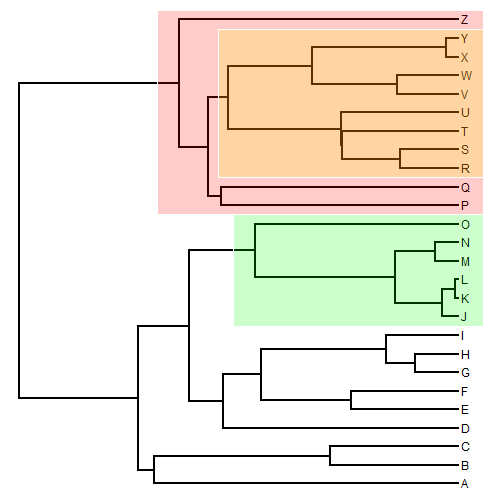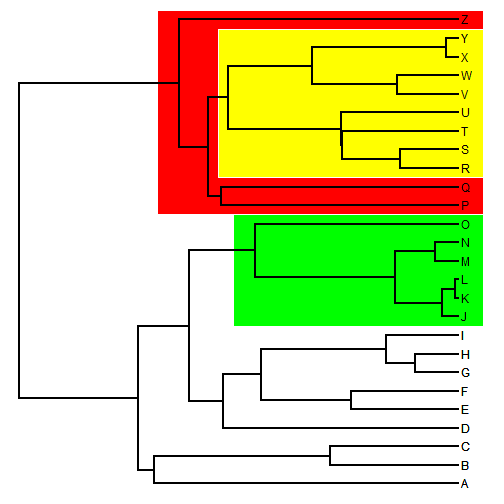## Friday, May 5, 2017

### Simple method to draw boxes around clades on a plotted tree in R

I just wrote a simple function for drawing a box around a clade. This needs a lot of refinement, but it works as follows:

``````cladebox<-function(tree,node,color=NULL,...){
if(is.null(color)) color<-make.transparent("yellow",0.2)
obj<-get("last_plot.phylo",envir=.PlotPhyloEnv)
h<-max(nodeHeights(tree))
parent<-tree\$edge[which(tree\$edge[,2]==node),1]
x0<-max(c(obj\$xx[node]+obj\$xx[parent])/2,obj\$xx[node]-0.05*h)
x1<-obj\$x.lim
dd<-getDescendants(tree,node)
y0<-min(range(obj\$yy[dd]))-0.5
y1<-max(range(obj\$yy[dd]))+0.5
polygon(c(x0,x1,x1,x0),c(y0,y0,y1,y1),col=color,
border=0)
}
library(phytools)
plotTree(tree)
``````If we want to use non-transparent colors, we can just add the tree backk on top of our boxes. For instance:

``````plotTree(tree)